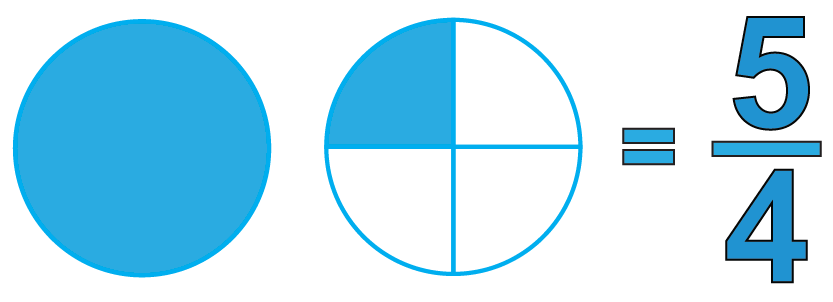Math Puzzles

# Free Math puzzles with answers on fractions

1.9k views

 1 Introduction 2 What Are the Parts of a Fraction? 3 Few examples on fraction 4 Summary 5 About Cuemath 6 Frequently Asked Questions (FAQs) on fractions 7 External References

29 December 2020

## Introduction

Why learning fractions is important?

Fractions are like that foundation. It's a much-needed part to keep up the walls later on. Without knowledge of fractions, you will not be able to do algebra. Each part of math is building on the previous part.

Where We use fractions in day to day life

• Math & Shopping
• Fractions
• Cooking
•  Grocery shopping
• Meal prep for the family
•  Fitness
• Jewelry
• Pizza for the kids
•  Money in general
• Gas tank
• Fast food
• Photography
• Time

Math puzzle for kids

Math puzzles help children not only gain a deeper insight into mathematical concepts but also strengthen their critical thinking skills. Keeping this in mind, we have designed a fun and engaging math puzzle that will help your child understand math in a fun way.

-Comprehend the language of fractions;

-Understand when a fraction is more or less than a half.

-Solve problems involving more than one criteria.

## What Are the Parts of a Fraction?

• All fractions consist of a numerator and a denominator.
• The denominator indicates how many parts the whole has been divided into.
• It is placed in the lower part of the fraction.
• The numerator indicates how many sections of the fraction are represented.
• It is placed in the upper part of the whole.

## Few examples on fraction

 Example 1

Milk is sold at $16 per gallon. Find the cost of $$6\frac{2}{5}$$ gallons of milk. Solution Cost of one gallon of milk =$16

Therefore, the cost of $$6\frac{2}{5}$$ gallons i.e. $$\frac{32}{5}$$ gallons will be

\begin{align} &= \frac{{32}}{5} \times 16 \\[0.2cm] &= 102.4 dollars \end{align}

 $$\therefore$$ The cost of $$6\frac{2}{5}$$ gallons of milk is \\$102.4
 Example 2

In a class of 48 students, $$\frac{1}{4}$$ of them watch cartoons.

How many students do not watch cartoons?

Solution

Total number of students = 48

Number of students who watch cartoons = $$\frac{1}{4} \times 48 = 12$$

Thus, the number of students who don't watch cartoons $$= 48 - 12 = 36$$

 $$\therefore$$ The number of students who do not watch cartoons is 36
 Example 3

The snowfall during the first three months in winter was 30.5 inches, 45.25 inches, and 25.25 inches.

What was the total amount of snow in these months?

Solution

\begin{align} \text{ Total amount of snow for three months} \\&= 30.5 + 45.25 + 25.25 \\[0.2cm] &= \frac{{305}}{{10}} + \frac{{452.5}}{{10}} + \frac{{252.5}}{{10}} \\[0.2cm] & = \frac{{305 + 452.5 + 252.5}}{{10}} \\[0.2cm] & = \frac{{1010}}{{10}} \\[0.2cm] & = 101inches \\[0.2cm] \end{align}

 $$\therefore$$ The total amount of snow in 3 months was 101 inches
 Example 4

Laura spends $$1\frac{1}{4}$$ hours reading a book every day.

She read the entire book in 6 days.

How many hours did she take to read the entire book?

Solution

Number of hours Laura reads every day =  $$1\frac{1}{4}$$ hours = $$\frac{5}{4}$$ hrsShe finishes reading the book in 6 days.

Number of hours she took to read the entire book $$= 6 \times \frac{5}{4} = \frac{{30}}{4} = 7\frac{2}{4}$$ hours

 $$\therefore$$ Laura took  $$7\frac{2}{4}$$ hours to read entire book

## Summary

Mini-lesson targeted the fascinating concept of Fractions. The math journey around Fractions starts with what a student already knows, and goes on to creatively crafting a fresh concept in the young minds. Done in a way that not only it is relatable and easy to grasp, but also will stay with them forever. Here lies the magic with Cuemath.

Cuemath, a student-friendly mathematics platform, conducts regular Online Live Classes for academics and skill-development and their Mental Math App, on both iOS and Android, is a one-stop solution for kids to develop multiple skills. Understand the Cuemath fee Structure and sign up for a free trial.

## What is 0.125 as a fraction?

0.125 as fraction can be written as, $$\frac{{125}}{{1000}} = \frac{5}{{40}} = \frac{1}{8}$$

## What is the unit fraction of 5/8?

The unit fraction $$\frac58$$ is the same as the unit fraction $$\frac18$$ represented five times.

## What are the most common fractions?

The most common fractions are $$\frac14$$, $$\frac12$$, and $$\frac34$$.